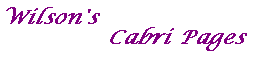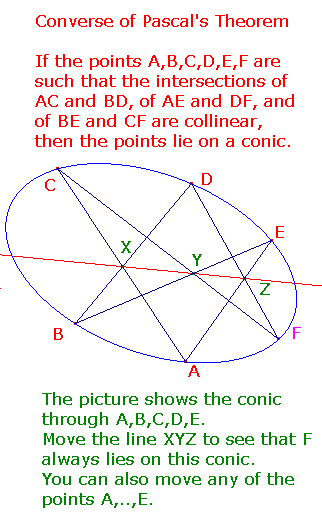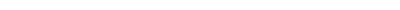Pascal's Theorem states that if A,..,F lie on a conic, then X,Y and Z are collinear. The converse is quite easy to deduce from the theorem. Outline of a proof: Consider the point F' where CY cuts the conic. Apply Pascal's Theorem to A,B,C,D,E,F' to see that DF' must pass through Z (the intersection of AE and XY). Then conclude that F=F'. This allows us to obtain any number of points on the conic defined by five fixed points A,B,C,D,E. The Braikenridge-Maclaurin Construction Let AC and BD meet in X. Draw any line L through meeting BE and AE. Suppose L cuts BE at Y and AE at Z. Let CY and DZ meet at F. By the Converse of Pascal's Theorem, F lies on the conic. Of course the conic is the locus of points F so obtained. The Cabri figure conpascal2.fig illustrates this. If you don't have Cabri, there is a screen shot here. Tell me about Pascal about Braikenridge about Maclaurin Download conpascal.figMain Cabri PageClassical Theorems